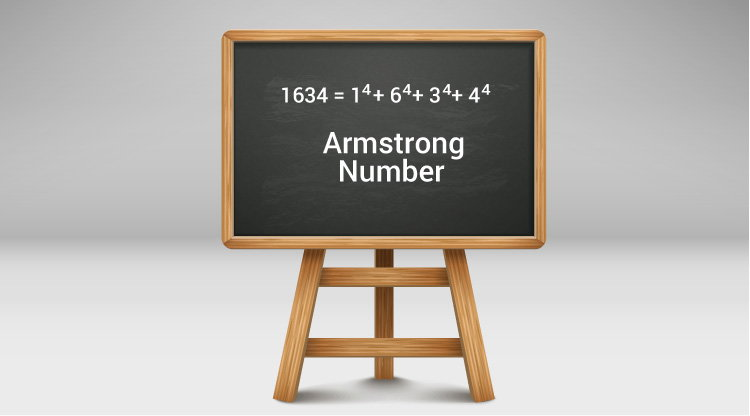# C Program to Display Armstrong Number Between Two Intervals

In this example, you will learn to find all Armstrong numbers between two integers entered by the user.To understand this example, you should have the knowledge of the following C programming topics:

A positive integer is called an Armstrong number (of order n) if

``````abcd... = an + bn + cn + dn + �
``````

In the case of an Armstrong number of 3 digits, the sum of cubes of each digit is equal to the number itself. For example, 153 is an Armstrong number because

```153 = 1*1*1 + 5*5*5 + 3*3*3
```

Before trying this program, learn how to check whether an integer is an Armstrong number or not.

## Armstrong Numbers Between Two Integers

``````#include <math.h>
#include <stdio.h>
int main() {
int low, high, i, temp1, temp2, rem, n = 0;
float result = 0.0;
printf("Enter two numbers(intervals): ");
scanf("%d %d", &low, &high);
printf("Armstrong numbers between %d and %d are: ", low, high);

for (i = low + 1; i < high; ++i) {
temp2 = i;
temp1 = i;

// number of digits calculation
while (temp1 != 0) {
temp1 /= 10;
++n;
}

// result contains sum of nth power of its digits
while (temp2 != 0) {
rem = temp2 % 10;
result += pow(rem, n);
temp2 /= 10;
}

// check if i is equal to the sum of nth power of its digits
if ((int)result == i) {
printf("%d ", i);
}

// resetting the values
n = 0;
result = 0;
}

return 0;
}
``````

Output

```Enter two numbers(intervals): 999
9999
Armstrong numbers between 999 and 9999 are: 1634 8208 9474
```